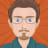Published 2021-09-25 by Michael Ward

# Exclusively required method parameters with TypeScriptUsing TypeScript to enforce interfaces and data types in JavaScript applications has become a really important part of development, easing the path to robust data handling within applications. In many cases TypeScript is simple to use, but has a lot of options to cover more complex scenarios.

In this article, I am going to look at method signatures and how TypeScript handles the situation when mutually exclusive method parameters are required.

## The example method

``const myMethod = ({ param1, numberParam, arrayOfNumbersParam }) => ...``

As a simple example, we'll say that `param1` is a required `string` and that `numberParam` is an optional `number` and `arrayOfNumbersParam` is an optional array of numbers (`number[]`).

Enforcing this signature with TypeScript is simple using a function type :

``````// Function type
type MyMethodType = (params: { param1: string, numberParam?: number, arrayOfNumbersParam?: number[] }) => void

// Corresponding method signature
const myMethod: MyMethodType = ({ param1, numberParam, arrayOfNumbersParam }) => ...``````

This is great, but it isn't always the case that methods can be described this easily.

## A more complex example

Lets take the example method:

``const myMethod = ({ param1, numberParam, arrayOfNumbersParam }) => ...``

This method (theoretically) takes the `numberParam` and calculates an array of numbers from that value, or alternatively it takes an array of numbers passed via the `arrayOfNumbersParam` and uses that.

One of the two parameters must be supplied, but not both. How does TypeScript allow us to enforce this type of signature? You may not be surprised to find that there's more than one way, and below I describe two that I like.

Function overloading  allows the definition of multiple signatures for a single function. This is very helpful for this use case.

Here's how the function type works for our scenario:

``````type MyMethodType = {
({ param1: string, numberParam: number }) => void
({ param1: string, arrayOfNumbersParam: number[] }) => void
}

const myMethod: MyMethodType = ({ param1, numberParam, arrayOfNumbersParam) => ...``````

The two signatures describe the two scenarios for the function call and TypeScript will flag any attempt to call the method using both `numberParam` and `arrayOfNumbersParam` arguments.

``````// Acceptable
myFunction({ param1: 'test', numberParam: 2 })

// Acceptable
myFunction({ param1: 'test', arrayOfNumbersParam: [2, 3, 4] })

// TypeScript error
myFunction({ param1: 'test', numberParam: 2, arrayOfNumbersParam: [2, 3, 4] })``````

However, if you're following along, you may have noticed that the type defined has a problem - neither signature, both of which have two parameters, match the function definition which has three parameters. TypeScript correctly flags this as an error.

To fix this issue, we must add a third parameter to each of the signatures. TypeScript has a handy `never` type that allows us to specify that the third parameter should never be used.

``````type MyMethodType = {
({ param1: string, numberParam: number, arrayOfNumbersParam?: never }) => void
({ param1: string, arrayOfNumbersParam: number[], numberParam?:never }) => void
}

const myMethod: MyMethodType = ({ param1, numberParam, arrayOfNumbersParam) => ...``````

The type definition now accurately describes the function definition and its' intended usage.

## A DRYer way

Whilst describing complex method or function signature with function overloading may be the right way to go in many situations, I do have a problem with this particular implementation.

The `param1` and `return` types are the same for both signatures, and it does not feel very DRY  to repeat this and to introduce the potential to have inconsistent and incorrect function type definitions.

Rather than use function overloads, there is an alternative.

Firstly, define the common aspects of the two signatures:

`````` type MyMethodType = {
(params: { param1: string }) => void
}

const myMethod: MyMethodType = ({ param1, numberParam, arrayOfNumbersParam) => ...``````

Utilising TypeScript union types , the two alternate signatures can be added:

``````type myFunctionType = {
(
params: { param1: string } & (
| { numberParam: number; arrayOfNumbersParam?: never }
| { arrayOfNumbersParam: number[]; numberParam?: never }
)
): void
}

const myMethod: MyMethodType = ({ param1, numberParam, arrayOfNumbersParam) => ...``````

This method avoids repetition and fully meets the requirements of the method signature.